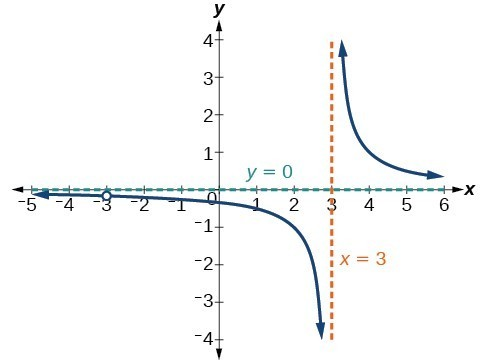## Find the domains of rational functions

A vertical asymptote represents a value at which a rational function is undefined, so that value is not in the domain of the function. A reciprocal function cannot have values in its domain that cause the denominator to equal zero. In general, to find the domain of a rational function, we need to determine which inputs would cause division by zero.

### A General Note: Domain of a Rational Function

The domain of a rational function includes all real numbers except those that cause the denominator to equal zero.

### How To: Given a rational function, find the domain.

1. Set the denominator equal to zero.
2. Solve to find the x-values that cause the denominator to equal zero.
3. The domain is all real numbers except those found in Step 2.

### Example 4: Finding the Domain of a Rational Function

Find the domain of $f\left(x\right)=\frac{x+3}{{x}^{2}-9}$.

### Solution

Begin by setting the denominator equal to zero and solving.

$\begin{cases} {x}^{2}-9=0 \hfill \\ \text{ }{x}^{2}=9\hfill \\ \text{ }x=\pm 3\hfill \end{cases}$

The denominator is equal to zero when $x=\pm 3$. The domain of the function is all real numbers except $x=\pm 3$.

### Analysis of the Solution

A graph of this function confirms that the function is not defined when $x=\pm 3$.Figure 8

There is a vertical asymptote at $x=3$ and a hole in the graph at $x=-3$. We will discuss these types of holes in greater detail later in this section.

### Try It 4

Find the domain of $f\left(x\right)=\frac{4x}{5\left(x - 1\right)\left(x - 5\right)}$.

Solution